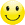## How to Fix the Wrong Subtotal Time:

I created a timesheet in MS Excel and wanted to show the total time I had worked over a week, (a filtered subtotal). It worked great when it was just a few hours, but gave the wrong figure when I started accumulating more hours?

Topics:

## How to Fix the Subtotal Formula not Showing the Correct Result (Time):

The Cause:

When you format time in Excel it tends to format as hh:mm:ss. This formating works on the 24 hour clock. So, when you go past 24 hours, Excel is looking to start the clock again. This can cause the error.

Time is very often formatted as:

hh:mm:ss (or similar)

The image below gives an example:

Note:

• To format a cell, right click the cell (or cells) and select ‘Format Cells…’

Using the wrong cell formatting can give you the wrong total value (sum):

It may be confusing trying to find the error… Because if the total sum is less than 24 hours, the subtotal will appear to work?

The Fix:

To get the correct ‘Total’ value when going above 24 Hrs, (using the formula =SUBTOTAL(function_num, ref1, …), we need to format the cell differently. To avoid the 24 hour formatting restriction of hh:mm:ss.

We can format the subtotal as:

[h]:mm;@

The image below gives an example:

Hope this helps…